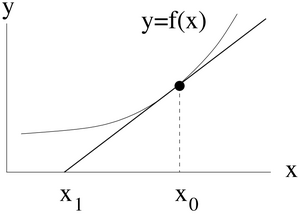# Science:Math Exam Resources/Courses/MATH102/December 2013/Question A 04

MATH102 December 2013
Other MATH102 Exams

### Question A 04

Shown in the figure below is a function and its tangent line at $x=x_{0}$.The tangent line intersects the $x$axis at the point $x=x_{1}$. The coordinate of the point $x_{1}$is

(a) $x_{1}=x_{0}+{\frac {f(x_{0})}{f'(x_{0})}}$(b) $\displaystyle x_{1}=x_{0}-f'(x_{0})(x-x_{0})$(c) $x_{1}=x_{0}-{\frac {f'(x_{1})}{f(x_{1})}}$(d) $x_{1}=x_{0}+{\frac {f'(x_{1})}{f(x_{1})}}$(e) $x_{1}=x_{0}-{\frac {f(x_{0})}{f'(x_{0})}}$Make sure you understand the problem fully: What is the question asking you to do? Are there specific conditions or constraints that you should take note of? How will you know if your answer is correct from your work only? Can you rephrase the question in your own words in a way that makes sense to you? If you are stuck, check the hints below. Read the first one and consider it for a while. Does it give you a new idea on how to approach the problem? If so, try it! If after a while you are still stuck, go for the next hint.

 Checking a solution serves two purposes: helping you if, after having used all the hints, you still are stuck on the problem; or if you have solved the problem and would like to check your work. If you are stuck on a problem: Read the solution slowly and as soon as you feel you could finish the problem on your own, hide it and work on the problem. Come back later to the solution if you are stuck or if you want to check your work. If you want to check your work: Don't only focus on the answer, problems are mostly marked for the work you do, make sure you understand all the steps that were required to complete the problem and see if you made mistakes or forgot some aspects. Your goal is to check that your mental process was correct, not only the result.Math Learning Centre A space to study math together. Free math graduate and undergraduate TA support. Mon - Fri: 11 am - 5 pm, Private tutor We can help to